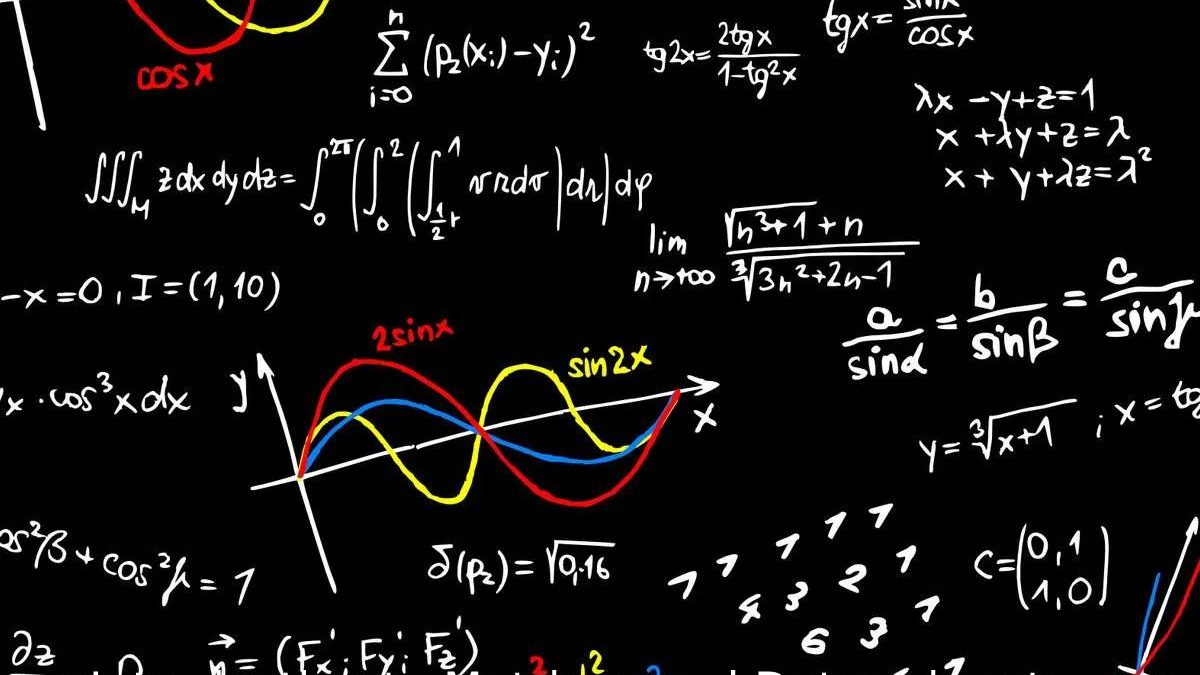### Trending Articles

29 Nov 2023# Introduction to Matrices and Determinants

A matrix is a set of numbers that have been arranged in columns and rows, forming a rectangular array of numbers. These numbers are named as entries or elements of the matrix. English mathematician James Sylvester first introduced the term ‘Matrix’ in the 19th century. The algebraic aspect of matrices was developed by his friend Arthur Caley, who was the first one to apply the concept of matrices to study linear equations. The concept of matrices and inverse of matrix is still very useful in the study of linear equations.

Matrices are widely used in computer graphics where matrices are used to represent the transformation and rotation of images. If there are y rows and z columns the matrix is said to be the “y X z” matrix. A matrix with b rows and b columns is called a square matrix of order n. While when we multiply two ordinary numbers m and n, mn is always equal to nm, the same is not true for the multiplication of matrices as it is not commutative.

Another term associated with linear equations is ‘Inverse of Matrix’. The inverse of a matrix is a type of matrix that, when multiplied with the given matrix yields the multiplicative identity. This method is used to find solutions to linear equations using the matrix inversion method. The inverse of the matrix is possible if the value of the determinant of the matrix is non-zero. If the determinant of a given matrix has a non-zero value and the inverse matrix can be calculated, such a matrix is called an invertible matrix. Therefore, the inverse of the matrix can exist only if the given matrix is a square matrix and the determinant of the said matrix is not equal to zero.

Let us look at some important terms related to the inverse of a matrix. These terms will prove helpful in doing calculations related to the inverse of the matrix and will make the concept clearer.

• Determinant – The determinant is a single unique value that represents a matrix. You need to refer to the row and column of the given matrix, to calculate the determinant.
• Cofactor – If you multiply the minor with -1 to the exponent of the summation of the row and column elements, you will get the cofactor of the element.
• Nonsingular Matrix – A nonsingular matrix is one whose determinant value is non-zero. Since it is possible to calculate the inverse of a non-singular matrix, it is also known as an invertible matrix.
• Singular Matrix – A singular matrix is the one whose determinant value is equal to zero. The inverse of a singular matrix cannot be calculated.

## What are the rules governing the row and column operations of a determinant?

Certain rules should be kept in mind while doing calculations about determinants:

• The value of the determinant does not change by interchanging the rows and columns.
• The sign of determinants will change if two rows or two columns are interchanged.
• The value of the determinant does not change by adding or subtracting the elements of a column or a row with the multiples of elements of another column or row.
• The value of the determinant is zero if the two rows or columns of a matrix have the same value.
• If you multiply the elements of a specific row or column by a constant the determinant is also multiplied by the same constant.
• When the elements of a row or column are expressed in the form of the sum of their elements, the determinants are also expressed as a sum of determinants.

## What are the various fields where the concept of the Invertible matrix is applied?

• Invertible matrices are used for the encryption of messages for security reasons. This has become a necessity in present times.
• Invertible matrices are used to transform images and play a significant role in Computer graphics in the 3D space.
• Coders and cryptographers use invertible matrices to decode messages. These are also used in programming algorithms for encryption.

If you want to go into further details, join one of the programs offered by Cuemath to get a clear understanding of the concept.